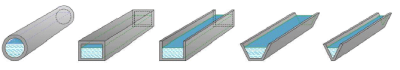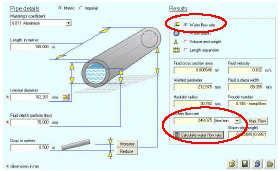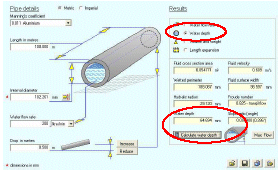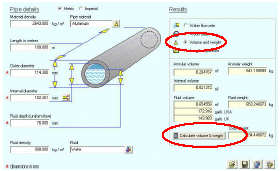# Channel Flow Calculator for part-full flow conditionsPipe Flow Advisor can calculate the water flow rate, water depth, volume of water, and weight of water flow in a channel. It can also calculate the length of pipe expansion under various conditions. The calculations for flow in channels can estimate:

• Flow Rate of Water in Part full pipes.
• Flow Rate of Water in Part full rectangular sections.
• Flow Rate of Water in Rectangular channels.
• Flow Rate of Water in Flat bottomed channels (with sloping sides).
• Flow Rate of Water in Vee channels.Examples of channel flow calculations are given below.

## Water Channel Flow Rate CalculationSelect the 'Water flow rate' option.
Select a Manning coefficient for the pipe material.
Enter pipe length, diameter, fluid depth & vertical drop
Choose the flow rate units required.

Click to calculate water flow rate in the channel.

## Water Depth CalculationSelect the 'Water Depth' option.
Select a Manning coefficient for the pipe material.
Enter pipe length, diameter, fluid depth & vertical drop

Click to calculate the depth of water in the channel.
Note: The maximum flow rate through a circular channel occurs when the water depth is approximately 93.8% of the internal diameter of the pipe. Select 'Max Flow' to calculate the maximum flow possible.

## Volume & Weight CalculationSelect the 'Volume and Weight' option.
Enter pipe length, inner & outer diam, & fluid depth.
Select or set the pipe material density.
Select or set the fluid density.

Click to 'Calculate Volume & Weight'.

## Channel Length Expansion CalculationSelect the 'Length Expansion' option.
Specify current pipe length.
Choose the change in temperature.

Click ot 'Calculate Pipe Length Expansion'.

Next: Weir Flow Calculator

Secure Online PaymentsPipe Flow Software: Piping design, Pressure drop calculator, Flow rate calculator, Pump head calculations, Pump selection software.  Copyright © Pipe Flow Software 1997-2023